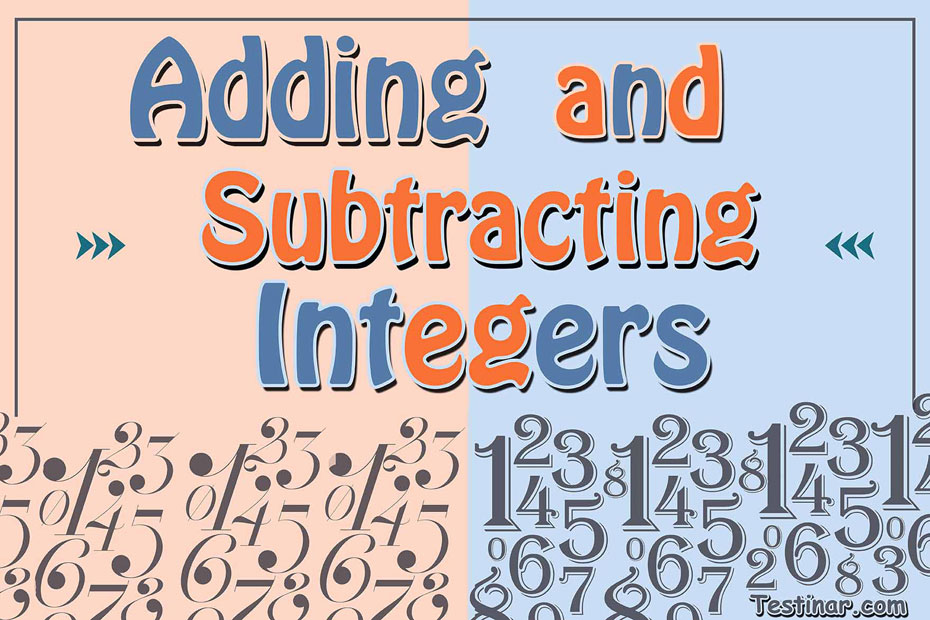## How to Add and Subtract Integers

The term integers represent the meaning “intact” or “whole”. So, you can generally refer an integer as a whole number, except integers can be negative also!

What is an Integer?

An integer is a whole number (not any decimal or fraction numbers) which can be zero, positive or negative numbers. Some examples of integers can be $$7 \ , \ 3 \ , \ 0 \ , \ -5 \ , \ -15$$, etc. Moreover, we can represent integers by the denotation $$Z$$ which comprises of:
Positive Integers: As the name suggests, any integer that is greater than zero is termed as a positive integer.
Negative Integer:  As from the name, any integer that is less than zero is termed as a negative integer.
Zero: Zero is neither a positive integer or a negative integer. It is just a whole number.
So, we can write $$Z = \{…… -5 \ , \ -4 \ , \ -3 \ , \ -2 \ , \ -1 \ , \ 0 \ , \ 1 \ , \ 2 \ , \ 3 \ , \ 4 \ , \ 5 \ , \ ……\}$$
Also, we can place all integers on a number line where the negative ones are placed on the left of “$$0$$” and the positive ones on the right. Moreover, we can perform the $$4$$ basic mathematic properties with integers. They are:

• Subtraction
• Multiplication
• Division

We often see that negative integers are always written as $$-5 \ , \ -9$$, and so on. But it is not generally considered necessary to write positive integers like $$+5 \ , \ +9$$, and so on. So, when we write just $$5$$, we mean $$+5$$.
Another thing to note is, that an absolute value of any integer is always positive. So, $$\lvert -6 \rvert = 6$$ and $$\lvert 6 \rvert$$  is also $$6$$.

How to Add and Subtract Integers

• In the first case, if two integers have the same sign (either both are positive or both are negative), add up those integers and put the common sign.
• In the second case, if two integers have an opposite sign (one is positive and the other one negative), then subtract them and put the sign of the bigger number.

Example:

• $$-12 +13 = +1$$
• $$-12 -13 = -25$$
• $$12 – 13 = -1$$ and so on.

### Exercises for Adding and Subtracting Integers

1) $$(-48) \ + \ (-27) \ =$$

2) $$(-34) \ + \ (-27) \ =$$

3) $$10 \ + \ (-26) \ =$$

4) $$(-19) \ + \ (3) \ =$$

5) $$(-26) \ + \ (-27) \ =$$

6) $$(-12) \ + \ (-13) \ =$$

7) $$(-41) \ + \ (-23) \ =$$

8) $$3 \ + \ (-22) \ =$$

9) $$25 \ + \ (-21) \ =$$

10) $$32 \ + \ (-20) \ =$$

1) $$(-48) \ + \ (-27) \ = \color{red}{-75}$$
Solution
Both numbers have the same sign (both are negative ), therefore add them together: $$48 + 27=75$$,
and then put the negative sign:$$-75$$
2) $$(-34) \ + \ (-27) \ = \color{red}{-61}$$
Solution
Both numbers have the same sign (both are negative ), therefore add them together: $$34 + 27=61$$,
and then put the negative sign:$$-61$$
3) $$10 \ + \ (-26) \ = \color{red}{-16}$$
Solution
Two numbers don’t have the same sign (one is negative and the other is positive), you must subtract them $$26 - 10=16$$,
and then put the bigger number sign, $$-16$$
4) $$(-19) \ + \ (3) \ = \color{red}{-16}$$
Two numbers don’t have the same sign (one is negative and the other is positive), you must subtract them $$19 - 3=16$$,
and then put the bigger number sign, $$-16$$
5) $$(-26) \ + \ (-27) \ = \color{red}{-53}$$

6) $$(-12) \ + \ (-13) \ = \color{red}{-25}$$

7) $$(-41) \ + \ (-23) \ = \color{red}{-64}$$

8) $$3 \ + \ (-22) \ = \color{red}{-19}$$

9) $$25 \ + \ (-21) \ = \color{red}{4}$$

10) $$32 \ + \ (-20) \ = \color{red}{12}$$

## Add and Subtract Integers Practice Quiz

### PSAT Math for Beginners

$24.99$14.99

### Prepare for the ALEKS Math Test in 7 Days

$19.99$12.99

### The Most Comprehensive GED Math Preparation Bundle

$79.99$34.99

### SSAT Upper Level Math Study Guide

$20.99$15.99